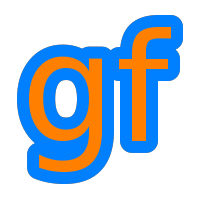Gamedev Framework (gf)  0.1.0 A C++11 framework for 2D games
gf::FractalNoise Member List

This is the complete list of members for gf::FractalNoise, including all inherited members.

 FractalNoise(std::function< double(double, double)> noise, double scale, std::size_t octaves=8, double lacunarity=2.0, double persistence=0.5, double dimension=1.0) gf::FractalNoise inline operator()(double x, double y) const gf::FractalNoise